# Solved Problems on Single Stage Transistor Amplifiers

### Q1 : Select a suitable value for the emitter bypass capacitor in Fig. 1 if the amplifier is to operate over a frequency range from 2 kHz to 10 kHz.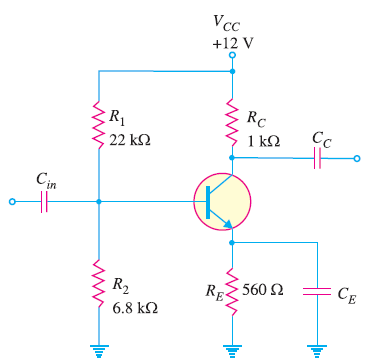Fig. 1

### Solution :

An amplifier usually handles more than one frequency. Therefore, the value of CE is so selected that it provides adequate bypassing for the lowest of all the frequencies. Then it will also be a good bypass ( XC ∝ 1/f ) for all the higher frequencies.

Suppose the minimum frequency to be handled by CE is fmin. Then CE is considered a good bypass if at fmin,### Q2 :For the transistor amplifier shown in Fig. 2, R1 = 10 kΩ, R2= 5 kΩ, RC = 1 kΩ, RE = 2 kΩ and RL= 1 kΩ. (i) Draw d.c. load line (ii) Determine the operating point (iii) Draw a.c. load line. Assume VBE = 0.7 V.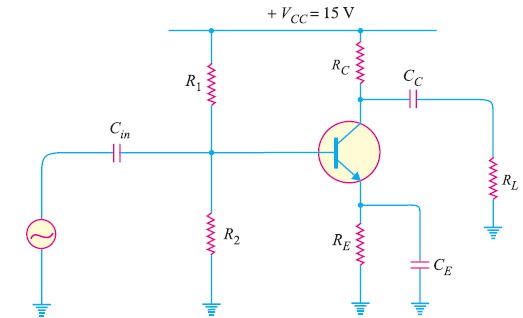Fig. 2

### Solution :

To draw d.c. load line, we require two end points i.e., maximum VCE point and maximum IC point.

Maximum  VCE =  VCC = 15 V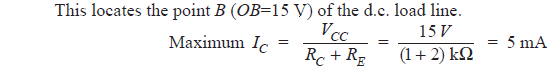This locates the point A (OA = 5 mA) of the d.c. load line. Fig. 3 shows the d.c. load line AB.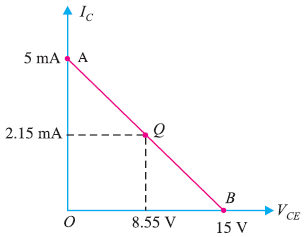Fig. 3

(ii) Operating point Q :

The Voltage across series combination of R1 and R2 is 15 V. Applying voltage divider theorem, voltage across
R2 = 5 V.

The voltage across R2 (= 5 kΩ ) is 5 V  i.e. V2 = 5 V.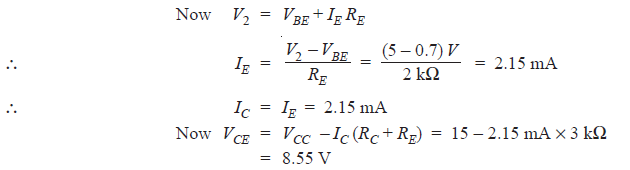∴ Operating point Q is 8.55 V, 2.15 mA. This is shown on the d.c. load line.

To draw a.c. load line, we require two end points viz. maximum collector-emitter voltage point and maximum collector current point when signal is applied.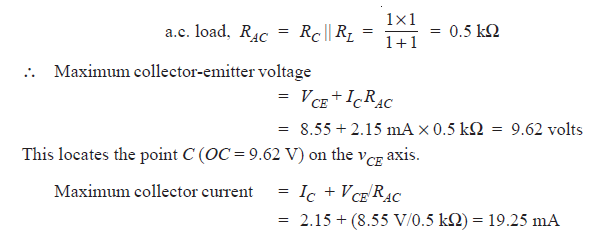This locates the point D (OD = 19.25mA) on the iC axis. By joining points C and D, a.c. load line CD is constructed as shown in Fig. 4.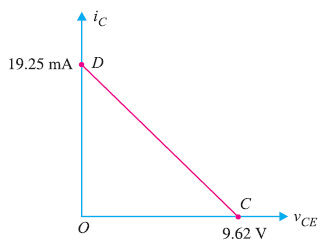Fig. 4

### Q3 : In the transistor amplifier shown in Fig. 5, RC = 10 kΩ, RL= 30 kΩ and VCC= 20V. The values R1 and R2 are such so as to fix the operating point at 10V, 1mA. Draw the d.c. and a.c. load lines. Assume RE is negligible.Fig. 5

### Solution :

For drawing d.c. load line, two end points such as maximum VCE point and maximum IC point are needed.

Maximum VCE = 20 V. This locates the point B (OB = 20V) of the d.c. load line on the VCE axis.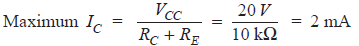This locates the point A (OA = 2 mA) on the IC axis. By joining points A and B, the d.c. load line AB is constructed as shown in Fig.6.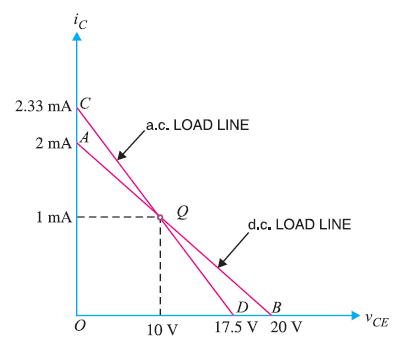Fig. 6

To draw a.c. load line, we require two end points viz maximum collector-emitter voltage point and maximum  collector current point when signal is applied.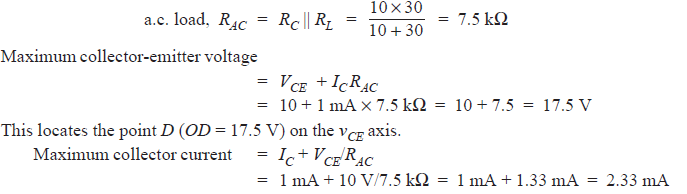This locates the point C (OC = 2.33 mA) on the iC axis. By joining points C and D, a.c. load line CD is constructed as shown in the above Fig. 6.

### Q4 : In the circuit shown in Fig. 7, find the voltage gain. Given that β = 60 and input resistance Rin = 1 kΩ.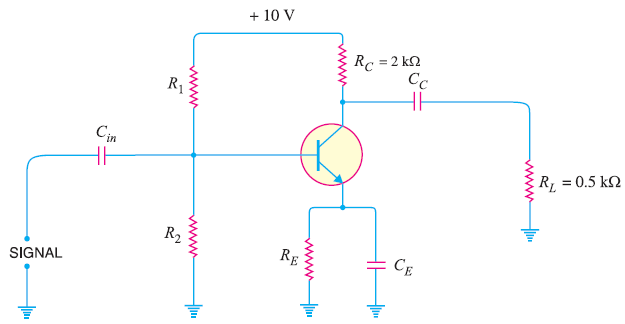Fig. 7

### Solution :

So far as voltage gain of the circuit is concerned, we need only RAC, β and Rin

### Solution :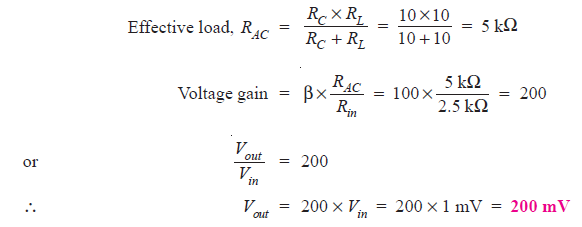### Solution :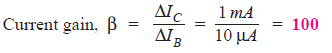(ii) input impedance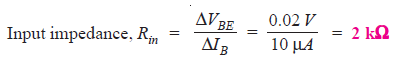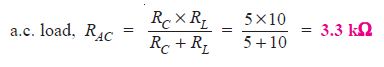(iv) voltage gain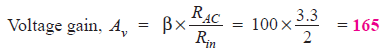(v) Power gain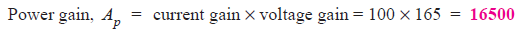### Q7 : In Fig. 9, the transistor has β = 50. Find the output voltage if input resistance Rin= 0.5 kΩ.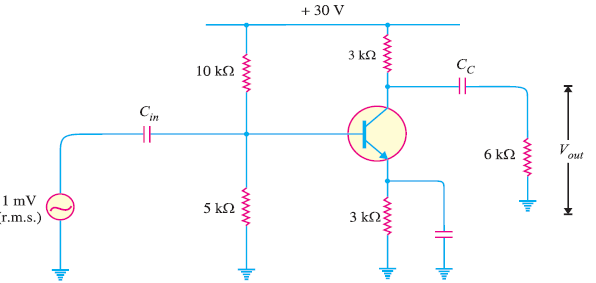Fig. 9

### Solution :

β = 50, Rin = 0.5 kΩ### Solution :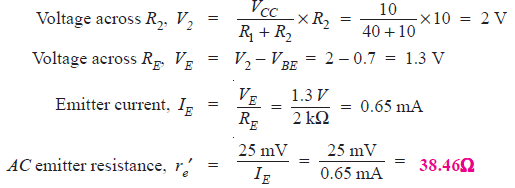### Q9 : For the amplifier circuit shown in Fig. 11, find the voltage gain of the amplifier with (i) CE connected in the circuit (ii) CE removed from the circuit.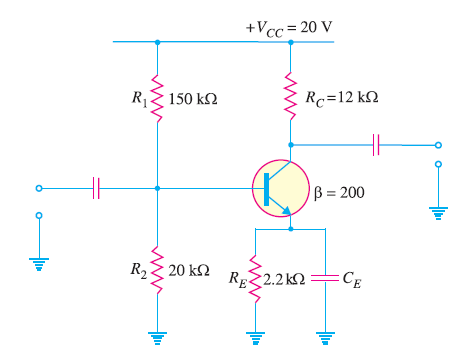Fig. 11

### Solution :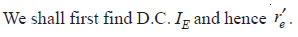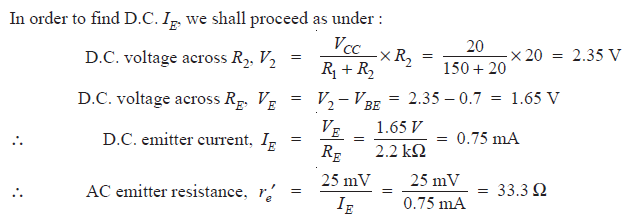(i) With CE connected :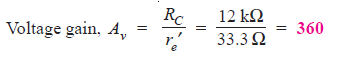(ii) Without CE :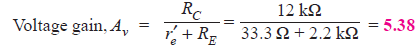### Q10 : For the circuit shown in Fig. 12, find (i) a.c. emitter resistance (ii) voltage gain (iii) d.c. voltage across both capacitors.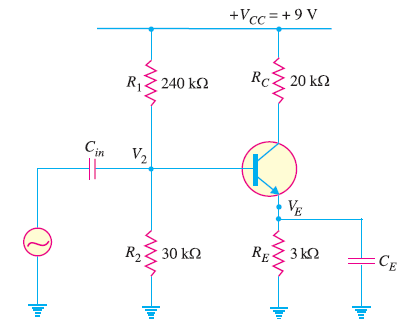Fig. 12

### Solution :

(i) In order to find a.c. emitter resistance re′ , we shall first find D.C. emitter current I. To find IE, we proceed as under: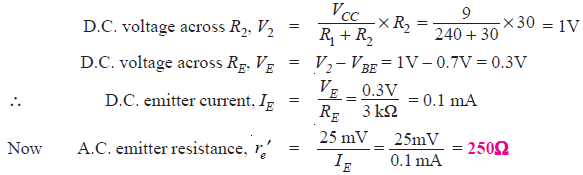(ii)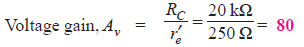(iii) The d.c. voltage across input capacitor is equal to the d.c. voltage at the base of the transistor which is V2 = 1V. Therefore, d.c. voltage across Cin is 1V.

Similarly, d.c. voltage across CE = d.c voltage at the emitter = VE = 0.3V.

### Q11 : For the circuit shown in Fig. 13, find (i) the d.c. bias levels (ii) d.c. voltages across the capacitors (iii) a.c. emitter resistance (iv) voltage gain and (v) state of the transistor.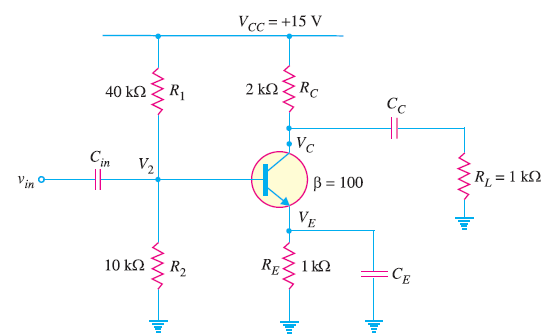Fig. 13

### Solution :

(i) D.C. bias levels :

The d.c. bias levels mean various d.c. currents and d.c. voltages.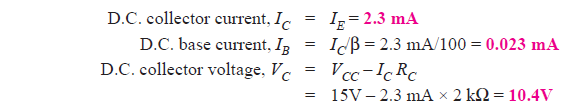Therefore, all d.c. bias levels stand calculated.

(ii) d.c. voltages across the capacitors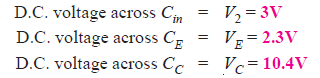(iii) a.c. emitter resistance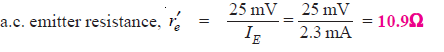(v) state of the transistor

As calculated above, VC = 10.4V and VE = 2.3V. Since VC > VE, the transistor is in active state.

### Solution :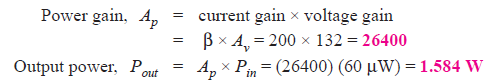### Q13. For the circuit shown in Fig. 14, determine (i) the current gain (ii) the voltage gain and (iii) the power gain. Neglect the a.c. emitter resistance for the transistor.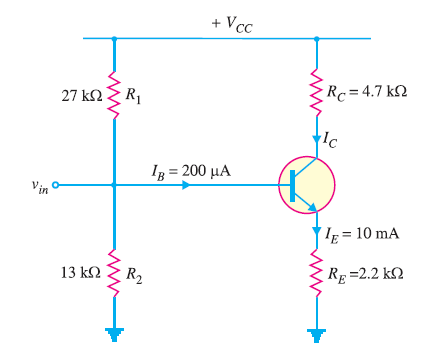Fig. 14

### Solution :

In most practical circuits, the value of a.c. emitter resistance re′ for the transistor is generally quite small as compared to RE and can be neglected in circuit calculations with reasonable accuracy.

(i)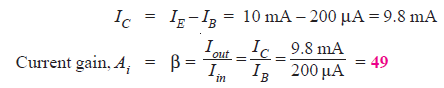(ii)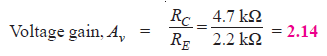(iii)### Q14. Determine the input impedance of the amplifier circuit shown in Fig. 15.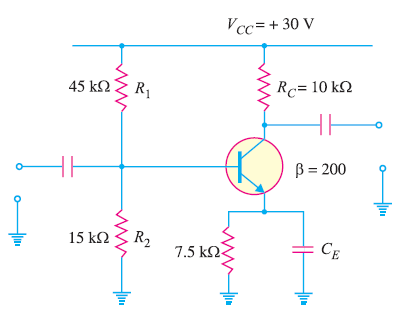Fig. 15

### Solution :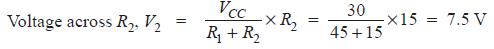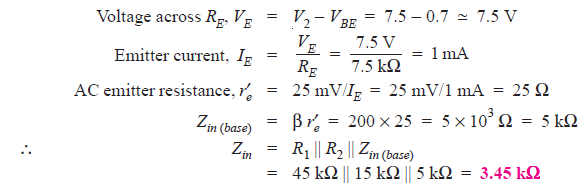### Solution :

Fig. 16 shows the equivalent circuit of the amplifier. Here A0 = 1000.Fig. 16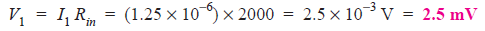### Solution :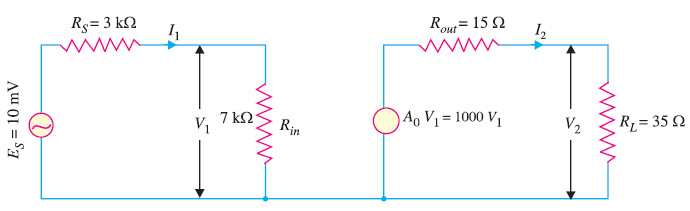Fig. 17

(i)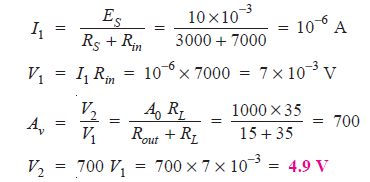(ii)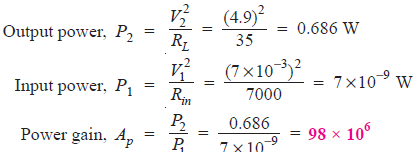### Solution :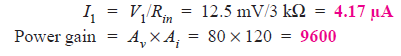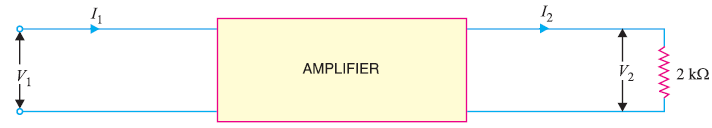Fig. 18